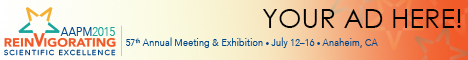# Program Information

## Parameter Optimization in Adaptive Region-Growing for Tumor Segmentation in PETS Tan

## Presentations

SU-C-9A-1 Sunday 1:00PM - 1:55PM Room: 9A

Purpose: To design a reliable method to determine the optimal parameter in the adaptive region-growing (ARG) algorithm for tumor segmentation in PET.

Methods: The ARG uses an adaptive similarity criterion m - fσ ≤ I_PET ≤ m + fσ, so that a neighboring voxel is appended to the region based on its similarity to the current region. When increasing the relaxing factor f (f ≥ 0), the resulting volumes monotonically increased with a sharp increase when the region just grew into the background. The optimal f that separates the tumor from the background is defined as the first point with the local maximum curvature on an Error function fitted to the f-volume curve. The ARG was tested on a tumor segmentation Benchmark that includes ten lung cancer patients with 3D pathologic tumor volume as ground truth. For comparison, the widely used 42% and 50% SUVmax thresholding, Otsu optimal thresholding, Active Contours (AC), Geodesic Active Contours (GAC), and Graph Cuts (GC) methods were tested. The dice similarity index (DSI), volume error (VE), and maximum axis length error (MALE) were calculated to evaluate the segmentation accuracy.

Results: The ARG provided the highest accuracy among all tested methods. Specifically, the ARG has an average DSI, VE, and MALE of 0.71, 0.29, and 0.16, respectively, better than the absolute 42% thresholding (DSI=0.67, VE= 0.57, and MALE=0.23), the relative 42% thresholding (DSI=0.62, VE= 0.41, and MALE=0.23), the absolute 50% thresholding (DSI=0.62, VE=0.48, and MALE=0.21), the relative 50% thresholding (DSI=0.48, VE=0.54, and MALE=0.26), OTSU (DSI=0.44, VE=0.63, and MALE=0.30), AC (DSI=0.46, VE= 0.85, and MALE=0.47), GAC (DSI=0.40, VE= 0.85, and MALE=0.46) and GC (DSI=0.66, VE= 0.54, and MALE=0.21) methods.

Conclusions: The results suggest that the proposed method reliably identified the optimal relaxing factor in ARG for tumor segmentation in PET.

Funding Support, Disclosures, and Conflict of Interest: This work was supported in part by National Cancer Institute Grant R01 CA172638. The dataset is provided by AAPM TG211.

Contact Email: